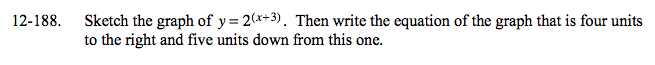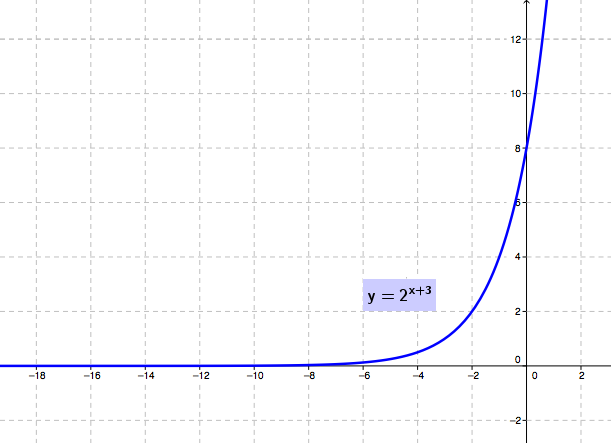### Home > A2C > Chapter 12 > Lesson 12.4.2 > Problem12-188

12-188.

Sketch the graph of y = 2(x+3). Then write the equation of the graph that is four units to the right and five units down from this one. Homework Help ✎Here is the original graph. What will the new one look like?